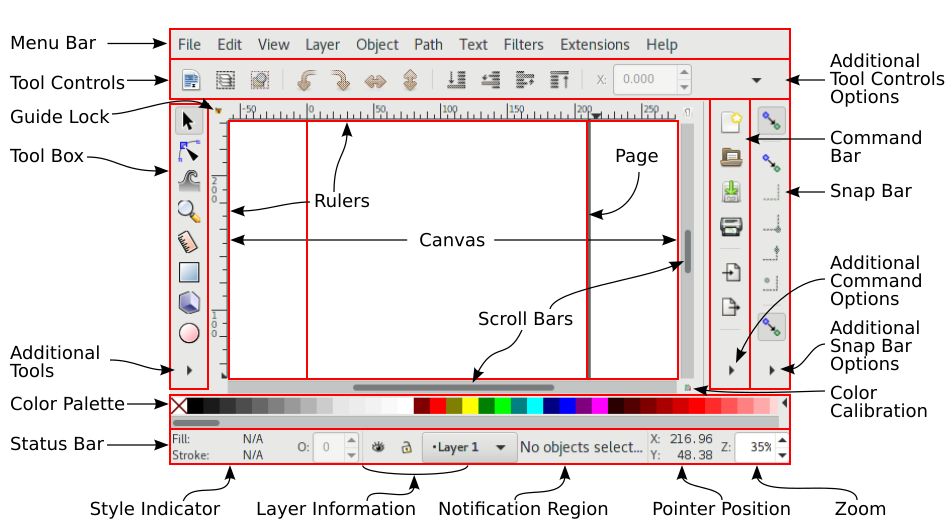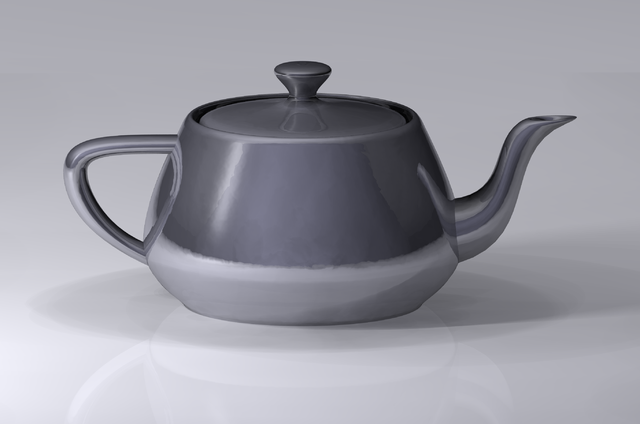# Viz - Stroke

stroke means painting along the outline of the object.

A stroke operator is a line along a path.

For each straight or curved segment in the path, the stroked line is centered on the segment with sides parallel to the segment.

Parameters:

• width
• opacity
• dasharray: controls the pattern of dashes (long, short, long,…)
• dashoffset: specifies the draw start point of a dash
• line cap
• line join
• miter limit

## Properties

### dasharray

Example with a SVG - (Straight) Line

``````line {
stroke: black;
stroke-width: 2;
}```
```
``````<svg>
<line stroke-dasharray="5, 5"              x1="10" y1="10" x2="190" y2="10" />
<line stroke-dasharray="5, 10"             x1="10" y1="30" x2="190" y2="30" />
<line stroke-dasharray="10, 5"             x1="10" y1="50" x2="190" y2="50" />
<line stroke-dasharray="5, 1"              x1="10" y1="70" x2="190" y2="70" />
<line stroke-dasharray="1, 5"              x1="10" y1="90" x2="190" y2="90" />
<line stroke-dasharray="0.9"               x1="10" y1="110" x2="190" y2="110" />
<line stroke-dasharray="15, 10, 5"         x1="10" y1="130" x2="190" y2="130" />
<line stroke-dasharray="15, 10, 5, 10"     x1="10" y1="150" x2="190" y2="150" />
<line stroke-dasharray="15, 10, 5, 10, 15" x1="10" y1="170" x2="190" y2="170" />
<line stroke-dasharray="5, 5, 1, 5"        x1="10" y1="190" x2="190" y2="190" />
</svg>```
```

### dashoffset

Example with a SVG - (Straight) Line

``````line {
stroke: black;
stroke-width: 2;
}```
```
``````<svg>
<line stroke-dasharray="50, 15"   stroke-dashoffset="00"          x1="10" y1="10" x2="190" y2="10" />
<line stroke-dasharray="50, 15"   stroke-dashoffset="10"          x1="10" y1="30" x2="190" y2="30" />
<line stroke-dasharray="50, 15"   stroke-dashoffset="20"          x1="10" y1="50" x2="190" y2="50" />
<line stroke-dasharray="50, 15"   stroke-dashoffset="30"          x1="10" y1="70" x2="190" y2="70" />
<line stroke-dasharray="50, 15"   stroke-dashoffset="40"          x1="10" y1="90" x2="190" y2="90" />
<line stroke-dasharray="50, 15"   stroke-dashoffset="50"          x1="10" y1="110" x2="190" y2="110" />
<line stroke-dasharray="50, 15"   stroke-dashoffset="60"          x1="10" y1="130" x2="190" y2="130" />
<line stroke-dasharray="50, 15"   stroke-dashoffset="70"          x1="10" y1="150" x2="190" y2="150" />
</svg>```
```

#### Animation

``````rect {
stroke: #ECDCC6;
stroke-width: 5;
stroke-dasharray: 600;
animation: dash 5s linear alternate infinite;
}

@keyframes dash {
from {
stroke-dashoffset: 0;
}
to {
stroke-dashoffset: 600;
}
}```
```
``````<svg>
<rect
x="5"
y="5"
width="200"
height="100"
fill="none"
rx="10"
ry="10"/>
</svg>```
```

## Documentation / Reference

Discover MoreGeometry - Shape (with transformation and notion)

in Geometry A shape is any list of line drawing commands, which strung together, describe a continuous line or closed shape that can be filled or stroked. Move, Line, Quadratic and Cubic...Inkscape - Stroke

stroke in Inkscape Hold Shift and select a color in the palette bar.SVG - Stroke

Stroke in Svg - stroke means painting along the outline of the object. The center width of a stroke is the edge of each shape. The circle with opacity The total rayon will be:Visualization - Painting / Rasterizing

paint renders pixels to screen. painting operators: stroke and fill.